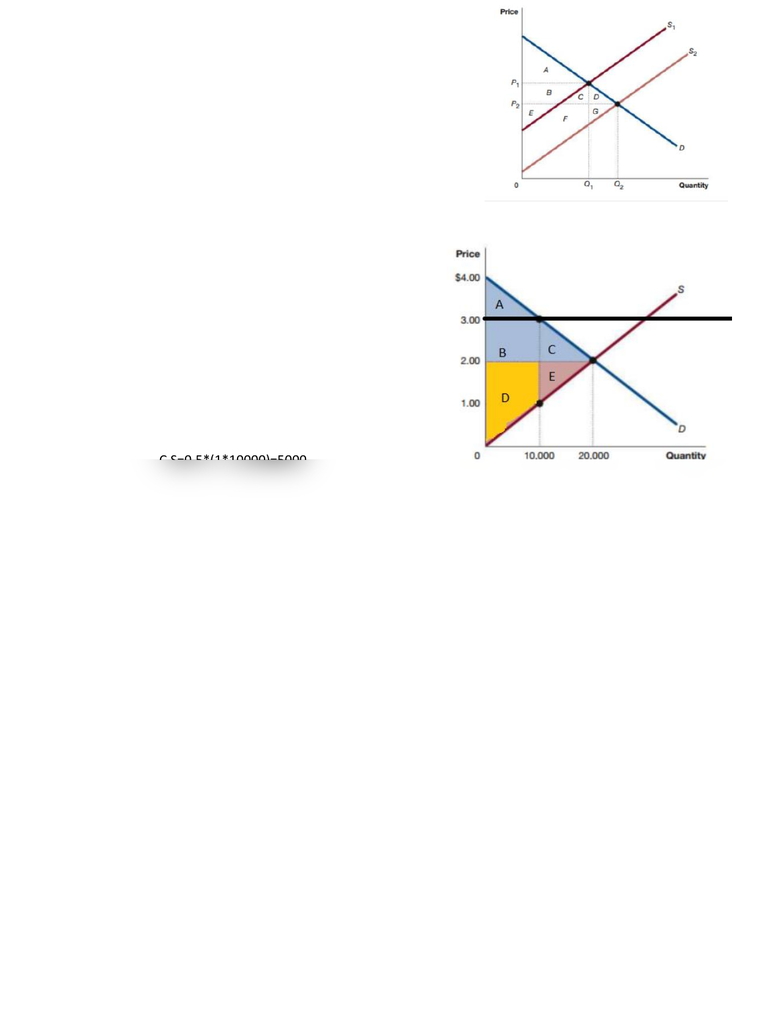Class Notes (1,100,000)
US (470,000)
UNH (600)
ECON (20)
Lecture 4

# ECON 402 Lecture 4: 4 chapter

Department
Economics
Course Code
ECON 402
Professor
William Crowley
Lecture
4

This preview shows half of the first page. to view the full 1 pages of the document.2.8
At the supply curve at S1 the consumer surplus is area A and
the supplier is the sum of areas B, C, D, E, F, and G. After the
supply curved shifted to the right and stays at S2 the
consumer surplus became the sum of the areas A, B, C, D and
the supplier surplus became the sum of the area E, F, G. Even
though the total surplus did not change, the consumer
surplus increased and the supplier surplus decreased.
4A.8
A. The deadweight lost is the sum of areas C and E. It is
equal to the sum of areas C and E.
D.L=0.5*(1*10000) + 0.5*(1*10000) =10000
B. The transfer of consumers surplus to producer
surplus is area B
TR=1*10000=10000
C. The producer surplus after the price floor is
imposed is the sum of areas D and B
P.S=0,5*(2+1)*10000+10000=25000
D. The consumer surplus after the price floor is
imposed is area A
C.S=0.5*(1*10000)=5000